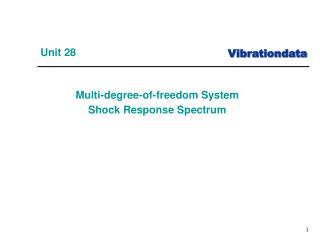DownloadDownload PresentationUnit 28

# Unit 28

Télécharger la présentation## Unit 28

- - - - - - - - - - - - - - - - - - - - - - - - - - - E N D - - - - - - - - - - - - - - - - - - - - - - - - - - -
##### Presentation Transcript

1. Unit 28 • Multi-degree-of-freedom System • Shock Response Spectrum

2. Introduction • The SRS can be extended to multi-degree-of-freedom systems • There are two options Modal transient analysis using synthesized waveform Approximation techniques using participation factors and normal modes

3. Two-dof System Subjected to Base Excitation Damping will be applied as modal damping

4. k2 ( x2 - x1 ) x1 m1 k1 ( x1 - y ) x2 m2 k2 (x2-x1) Free-Body Diagrams

5. Equation of Motion Assemble the equations in matrix form The equations are coupled via the stiffness matrix

6. Relative Displacement Substitution Define relative displacement terms as follows This works for some simple systems. Enforced acceleration method is required for other systems. The resulting equation of motion is

7. General Form where

8. Decoupling • Decouple equation of motion using eigenvalues and eigenvectors • The natural frequencies are calculated from the eigenvalues • The eigenvectors are the “normal modes” • Details given in accompanying reference papers

9. Proposed Solution Seek a harmonic solution for the homogeneous problem of the form where = the natural frequency (rad/sec) = modal coordinate vector or eigenvector

10. Solution Development The solution and its derivatives are Substitute into the homogeneous equation of motion

11. Generalized Eigenvalue Problem • Eigenvalues are calculated via where K is the stiffness matrix M is the mass matrix is the natural frequency (rad/sec) There is a natural frequency for each degree-of-freedom

12. Generalized Eigenvalue Problem (cont) • Calculate eigenvectors • The eigenvectors describe the relative displacement of the degrees-of-freedom for each mode • The overall motion of the system is a superposition of the individual modes for the case of free vibration • There is a corresponding mode shape for each natural frequency

13. Eigenvector Relationships Form matrix from eigenvectors Mass-normalize the eigenvectors such that (identity matrix) Then (diagonal matrix of eigenvalues)

14. Decouple Equation of Motion Define a modal displacement coordinate Substitute into the equation of motion Premultiply by Orthogonality relationships yields

15. Modified Equation of Motion • The equation of motion becomes • Now add damping matrix is the modal damping for mode i

16. Candidate Solution Methods, Time Domain • Runge-Kutta - becomes numerically unstable for “stiff” systems • Newmark-Beta - reasonably good – favorite of Structural Dynamics textbooks • Digital recursive filtering relationship - best choice but requires constant time step

17. Digital Recursive Filtering Relationship • The digital recursive filtering relationship is the same as that given in Webinar 17, SDOF Response to Applied Force - please review • The solution in physical coordinates is then

18. Participation Factors • Participation factors and effective modal mass values can be calculated from the eigenvectors and mass matrix • These factors represent how “excitable” each mode is • Might cover in a future Webinar, but for now please read: T. Irvine, Effective Modal Mass & Modal Participation Factors, Revision F, Vibrationdata, 2012

19. Participation Factors • Participation factors and effective modal mass values can be calculated from the eigenvectors and mass matrix • These factors represent how “excitable” each mode is • Might cover in a future Webinar, but for now please read: T. Irvine, Effective Modal Mass & Modal Participation Factors, Revision F, Vibrationdata, 2012

20. Participation Factors is the participation factor for mode i For the two-dof example in this unit

21. MDOF Estimation for SRS • ABSSUM – absolute sum method • SRSS – square-root-of-the-sum-of-the-squares • NRL – Naval Research Laboratory method

22. ABSSUM Method • Conservative assumption that all modal peaks occur simultaneously Pick D values directly off of Relative Displacement SRS curve where is the mass-normalized eigenvector coefficient for coordinate i and mode j These equations are valid for both relative displacement and absolute acceleration.

23. SRSS Method Pick D values directly off of Relative Displacement SRS curve These equations are valid for both relative displacement and absolute acceleration.

24. Example: Avionics Component & Base Plate m2 = 5 lbm Q=10 for both modes k2 = 4.6e+04 lbf/in Perform normal modes Transmissibility analysis m1 = 2 lbm k1 = 4.6e+04 lbf/in

25. vibrationdata > Structural Dynamics > Spring-Mass Systems > Two-DOF System Base Excitation

26. Normal Modes Results >> vibrationdata Natural Participation Effective Mode Frequency Factor Modal Mass 1 201.3 Hz 0.1311 0.0172 2 706.5 Hz 0.03063 0.0009382 modal mass sum = 0.01813 lbf sec^2/in (7.0 lbm) mass matrix 0.0052 0 0 0.0130 stiffness matrix 92000 -46000 -46000 46000 ModeShapes = 4.5606 13.1225 8.2994 -2.8844

27. Enter Damping

28. Transmissibility Analysis

29. Acceleration Transmissibility

30. Relative Displacement Transmissibility Relative displacement response is dominated by first mode.

31. SRS Base Input to Two-dof System SRS Q=10 Perform: Modal Transient using Synthesized Time History SRS Approximation srs_spec =[10 10; 2000 2000; 10000 2000]

32. Modal Transient Method, Synthesis File: srs2000G_accel.txt

33. Modal Transient Method, Synthesis (cont)

34. External File: srs2000G_accel.txt

35. Modal Transient Response Mass 1

36. Modal Transient Response Mass 2

37. SRS Approximation for Two-dof Example

38. Comparison Peak Accel (G) Both modes participate in acceleration response.

39. Comparison (cont) Peak RelDisp (in) Relative displacement results are closer because response is dominated by first mode.